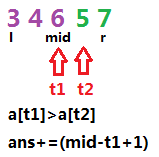CDQ分治【分治（真得头疼）

CDQ分治，传说中是一个♀神犇创造的算法

知识储备：分治

• 分治介绍
分而治之，将原问题不断划分成若干个子问题，直到子问题规模小到足以直接解决
子问题间互相独立且原问题形式相同，递归求解这些子问题，然后将各子问题的解合并得到原问题的解
• 一般步骤
• 划分 Divide
将原问题划分成若干子问题，子问题间互相独立且与原问题形式相同
• 解决 Conquer
递归解决子问题（递归是彰显分治优势的工具，仅仅进行一次分治策略也许看不出优势，但递归划分到子问题规模足够小，子问题的解可用常数时间解决）
• 合并 Merge
将各子问题的解合并得到原问题的解
• 时间复杂度
• 直观估计
• 分治由以上三部分构成，整体时间复杂度则由这三部分的时间复杂度之和构成
• 由于递归，最终的子问题变得极为简单，以至于其时间复杂度在整个分治策略上的比重微乎其微

ans+=(mid-t1+1)+1#include<cstdio>
#include<iostream>
#include<cstring>

using namespace std;

const int N=100010;
int a[N],b[N],n,ans=0;

void merge(int l,int r)
{
if (l==r) return;
int mid=(l+r)>>1;
merge(l,mid);
merge(mid+1,r);
int t1=l;
int t2=mid+1;
for (int i=l;i<=r;i++)
{
if ((t1<=mid&&a[t1]<=a[t2])||t2>r) {
b[i]=a[t1];
t1++;
}
else
{
b[i]=a[t2];
ans+=(mid-t1+1);
t2++;
}
}
for (int i=l;i<=r;i++) a[i]=b[i];
}

int main()
{
scanf("%d",&n);
for (int i=1;i<=n;i++) scanf("%d",&a[i]);
merge(1,n);
printf("%d",ans);
return 0;
} 

CDQ分治

CDQ分治是我们处理各类问题的重要武器

具体流程：

• 按照id（插入位置）归并排序
• 进行左区间的修改
• 统计右区间的询问

• 在合并的时候，我们只处理左区间的修改，只统计右区间的查询
因为左区间的修改一定可以影响右区间的查询
这就体现出了CDQ分治的基本思想了
• 我们把所有操作都记录到了一个数组中，所以数组的大小至少要开到500000*3
#include<cstdio>
#include<cstring>
#include<iostream>
#define ll long long

using namespace std;

const int N=5000010;
int n,m,totx=0,tot=0;     //totx是操作的个数,tot询问的编号

struct node{
int type,id;
ll val;
bool operator < (const node &a) const   //重载运算符,优先时间排序
{
if (id!=a.id) return id<a.id;
else return type<a.type;
}
};
node A[N],B[N];
ll ans[N];

void CDQ(int L,int R)
{
if (L==R) return;
int M=(L+R)>>1;
CDQ(L,M);
CDQ(M+1,R);
int t1=L,t2=M+1;
ll sum=0;
for (int i=L;i<=R;i++)
{
if ((t1<=M&&A[t1]<A[t2])||t2>R) //只修改左边区间内的修改值
{
if (A[t1].type==1) sum+=A[t1].val;   //sum是修改的总值
B[i]=A[t1++];
}
else                         //只统计右边区间内的查询结果
{
if (A[t2].type==3) ans[A[t2].val]+=sum;
else if (A[t2].type==2) ans[A[t2].val]-=sum;
B[i]=A[t2++];
}
}
for (int i=L;i<=R;i++) A[i]=B[i];
}

int main()
{
scanf("%d%d",&n,&m);
for (int i=1;i<=n;i++)
{
tot++;
A[tot].type=1; A[tot].id=i;            //修改操作
scanf("%lld",&A[tot].val);
}
for (int i=1;i<=m;i++)
{
int t;
scanf("%d",&t);
tot++;
A[tot].type=t;
if (t==1)
scanf("%d%lld",&A[tot].id,&A[tot].val);
else
{
int l,r;
scanf("%d%d",&l,&r);
totx++;
A[tot].val=totx; A[tot].id=l-1;    //询问的前一个位置
tot++; A[tot].type=3; A[tot].val=totx; A[tot].id=r;  //询问的后端点
}
}
CDQ(1,tot);
for (int i=1;i<=totx;i++) printf("%lld\n",ans[i]);
return 0;
}